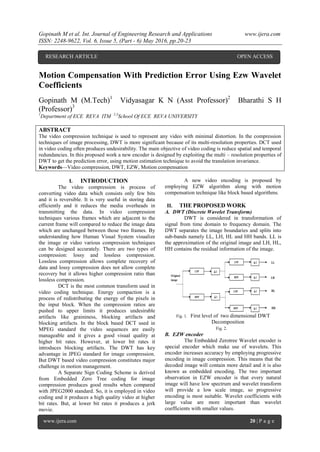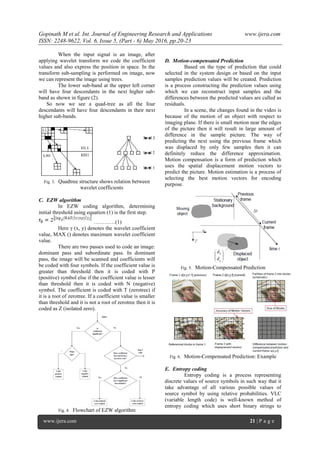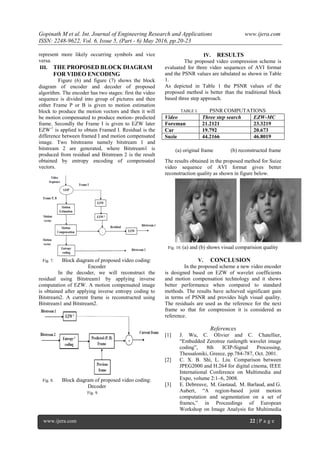Seu SlideShare está sendo baixado. ×

# Motion Compensation With Prediction Error Using Ezw Wavelet Coefficients

Anúncio
Anúncio
Anúncio
Anúncio
Anúncio
Anúncio
Anúncio
Anúncio
Anúncio
Anúncio
AnúncioCarregando em…3
×

1 de 4 Anúncio

# Motion Compensation With Prediction Error Using Ezw Wavelet Coefficients

The video compression technique is used to represent any video with minimal distortion. In the compression
techniques of image processing, DWT is more significant because of its multi-resolution properties. DCT used
in video coding often produces undesirability. The main objective of video coding is reduce spatial and temporal
redundancies. In this proposed work a new encoder is designed by exploiting the multi – resolution properties of
DWT to get the prediction error, using motion estimation technique to avoid the translation invariance.

The video compression technique is used to represent any video with minimal distortion. In the compression
techniques of image processing, DWT is more significant because of its multi-resolution properties. DCT used
in video coding often produces undesirability. The main objective of video coding is reduce spatial and temporal
redundancies. In this proposed work a new encoder is designed by exploiting the multi – resolution properties of
DWT to get the prediction error, using motion estimation technique to avoid the translation invariance.

Anúncio
Anúncio

### Motion Compensation With Prediction Error Using Ezw Wavelet Coefficients

1. 1. Gopinath M et al. Int. Journal of Engineering Research and Applications www.ijera.com ISSN: 2248-9622, Vol. 6, Issue 5, (Part - 6) May 2016, pp.20-23 www.ijera.com 20 | P a g e Motion Compensation With Prediction Error Using Ezw Wavelet Coefficients Gopinath M (M.Tech)1 Vidyasagar K N (Asst Professor)2 Bharathi S H (Professor)3 1 Department of ECE REVA ITM 2,3 School Of ECE REVA UNIVERSITY ABSTRACT The video compression technique is used to represent any video with minimal distortion. In the compression techniques of image processing, DWT is more significant because of its multi-resolution properties. DCT used in video coding often produces undesirability. The main objective of video coding is reduce spatial and temporal redundancies. In this proposed work a new encoder is designed by exploiting the multi – resolution properties of DWT to get the prediction error, using motion estimation technique to avoid the translation invariance. Keywords—Video compression, DWT, EZW, Motion compensation I. INTRODUCTION The video compression is process of converting video data which consists only few bits and it is reversible. It is very useful in storing data efficiently and it reduces the media overheads in transmitting the data. In video compression techniques various frames which are adjacent to the current frame will compared to reduce the image data which are unchanged between those two frames. By understanding how Human Visual System visualize the image or video various compression techniques can be designed accurately. There are two types of compression: lossy and lossless compression. Lossless compression allows complete recovery of data and lossy compression does not allow complete recovery but it allows higher compression ratio than lossless compression. DCT is the most common transform used in video coding technique. Energy compaction is a process of redistributing the energy of the pixels in the input block. When the compression ratios are pushed to upper limits it produces undesirable artifacts like graininess, blocking artifacts and blocking artifacts. In the block based DCT used in MPEG standard the video sequences are easily manageable and it gives a good visual quality at higher bit rates. However, at lower bit rates it introduces blocking artifacts. The DWT has key advantage in JPEG standard for image compression. But DWT based video compression constitutes major challenge in motion management. A Separate Sign Coding Scheme is derived from Embedded Zero Tree coding for image compression produces good results when compared with JPEG2000 standard. So, it is employed in video coding and it produces a high quality video at higher bit rates. But, at lower bit rates it produces a jerk movie. A new video encoding is proposed by employing EZW algorithm along with motion compensation technique like block based algorithms. II. THE PROPOSED WORK A. DWT (Discrete Wavelet Transform) DWT is considered in transformation of signal from time domain to frequency domain. The DWT separates the image boundaries and splits into sub-bands namely LL, LH, HL and HH bands. LL is the approximation of the original image and LH, HL, HH contains the residual information of the image. Fig. 1. First level of two dimensional DWT Decomposition Fig. 2. B. EZW encoder The Embedded Zerotree Wavelet encoder is special encoder which make use of wavelets. This encoder increases accuracy by employing progressive encoding in image compression. This means that the decoded image will contain more detail and it is also known as embedded encoding. The two important observation in EZW encoder is that every natural image will have low spectrum and wavelet transform will provide a low scale image, so progressive encoding is most suitable. Wavelet coefficients with large value are more important than wavelet coefficients with smaller values. RESEARCH ARTICLE OPEN ACCESS
2. 2. Gopinath M et al. Int. Journal of Engineering Research and Applications www.ijera.com ISSN: 2248-9622, Vol. 6, Issue 5, (Part - 6) May 2016, pp.20-23 www.ijera.com 21 | P a g e When the input signal is an image, after applying wavelet transform we code the coefficient values and also express the position in space. In the transform sub-sampling is performed on image, now we can represent the image using trees. The lower sub-band at the upper left corner will have four descendants in the next higher sub- band as shown in figure (2). So now we see a quad-tree as all the four descendants will have four descendants in their next higher sub-bands. Fig. 3. Quadtree structure shows relation between wavelet coefficients C. EZW algorithm In EZW coding algorithm, determining initial threshold using equation (1) is the first step. …………(1) Here γ (x, y) denotes the wavelet coefficient value, MAX () denotes maximum wavelet coefficient value. There are two passes used to code an image: dominant pass and subordinate pass. In dominant pass, the image will be scanned and coefficients will be coded with four symbols. If the coefficient value is greater than threshold then it is coded with P (positive) symbol else if the coefficient value is lesser than threshold then it is coded with N (negative) symbol. The coefficient is coded with T (zerotree) if it is a root of zerotree. If a coefficient value is smaller than threshold and it is not a root of zerotree then it is coded as Z (isolated zero). Fig. 4. Flowchart of EZW algorithm D. Motion-compensated Prediction Based on the type of prediction that could selected in the system design or based on the input samples prediction values will be created. Prediction is a process constructing the prediction values using which we can reconstruct input samples and the differences between the predicted values are called as residuals. In a scene, the changes found in the video is because of the motion of an object with respect to imaging plane. If there is small motion near the edges of the picture then it will result in large amount of difference in the sample picture. The way of predicting the next using the previous frame which was displaced by only few samples then it can definitely reduce the difference approximation. Motion compensation is a form of prediction which uses the spatial displacement motion vectors to predict the picture. Motion estimation is a process of selecting the best motion vectors for encoding purpose. Fig. 5. Motion-Compensated Prediction Fig. 6. Motion-Compensated Prediction: Example E. Entropy coding Entropy coding is a process representing discrete values of source symbols in such way that it take advantage of all various possible values of source symbol by using relative probabilities. VLC (variable length code) is well-known method of entropy coding which uses short binary strings to
3. 3. Gopinath M et al. Int. Journal of Engineering Research and Applications www.ijera.com ISSN: 2248-9622, Vol. 6, Issue 5, (Part - 6) May 2016, pp.20-23 www.ijera.com 22 | P a g e represent more likely occurring symbols and vice versa. III. THE PROPOSED BLOCK DIAGRAM FOR VIDEO ENCODING Figure (6) and figure (7) shows the block diagram of encoder and decoder of proposed algorithm. The encoder has two stages: first the video sequence is divided into group of pictures and then either Frame P or B is given to motion estimation block to produce the motion vectors and then it will be motion compensated to produce motion- predicted frame. Secondly the Frame I is given to EZW later EZW-1 is applied to obtain Framed I. Residual is the difference between framed I and motion compensated image. Two bitstreams namely bitstream 1 and bitstream 2 are generated, where Bitstream1 is produced from residual and Bitstream 2 is the result obtained by entropy encoding of compensated vectors. Fig. 7. Block diagram of proposed video coding: Encoder In the decoder, we will reconstruct the residual using Bitstream1 by applying inverse computation of EZW. A motion compensated image is obtained after applying inverse entropy coding to Bitstream2. A current frame is reconstructed using Bitstream1 and Bitstream2. Fig. 8. Block diagram of proposed video coding: Decoder Fig. 9. IV. RESULTS The proposed video compression scheme is evaluated for three video sequences of AVI format and the PSNR values are tabulated as shown in Table 1. As depicted in Table 1 the PSNR values of the proposed method is better than the traditional block based three step approach. TABLE I. PSNR COMPUTATIONS Video Three step search EZW-MC Foreman 21.2121 23.3219 Car 19.792 20.673 Suzie 44.2166 46.8019 The results obtained in the proposed method for Suize video sequence of AVI format gives better reconstruction quality as shown in figure below. Fig. 10. (a) and (b) shows visual comparision quality V. CONCLUSION In the proposed scheme a new video encoder is designed based on EZW of wavelet coefficients and motion compensation technology and it shows better performance when compared to standard methods. The results have achieved significant gain in terms of PSNR and provides high visual quality. The residuals are used as the reference for the next frame so that for compression it is considered as reference. References  J. Wu, C. Olivier and C. Chatellier, ―Embedded Zerotree runlength wavelet image coding‖, 8th ICIP-Signal Processing, Thessaloniki, Greece, pp.784-787, Oct. 2001.  C. X. B. Shi, L. Liu. Comparison between JPEG2000 and H.264 for digital cinema, IEEE International Conference on Multimedia and Expo, volume 2:1–6, 2008.  E. Debreuve, M. Gastaud, M. Barlaud, and G. Aubert, ―A region-based joint motion computation and segmentation on a set of frames,‖ in Proceedings of European Workshop on Image Analysis for Multimedia (a) original frame (b) reconstructed frame
4. 4. Gopinath M et al. Int. Journal of Engineering Research and Applications www.ijera.com ISSN: 2248-9622, Vol. 6, Issue 5, (Part - 6) May 2016, pp.20-23 www.ijera.com 23 | P a g e International Services (WIAMIS), Montreux, Swiss, Apr. 2005.  S. Boltz, E. Debreuve, and M. Barlaud, ―A joint motion segmentation algorithm for video coding‖, in Proceedings of EUSIPCO, Antalya, Turkey, 2005.  J. M. Shapiro,, ―Embedded Image Coding Using Zerotrees of Wavelet Coefficients‖, IEEE trans. on Signal Processing,Vol.41, No.12, pp.34453462, Dec. 1993.  W. Hassen, J.Mbainaibeye, N. Ellouze and C. Olivier ―Compression vidéo couleur basée sur le codage entropique du signe des coefficients en ondelettes en utilisant la compensation de mouvement‖, proceedings of Conférence internationale sur le Traitement et Analyse de l’Information, Méthodes et Applications TAIMA’09 pp.515-522, May 2009.  G. J.Sullivan and T. Wiegand, ―Video Compression-From Concepts to the H.264/AVC Standard‖, in Proceedings of IEEE. 2005  T. Wiegand, G. J. Sullivan, G. Bjøntegaard and A. Luthra, ―Overview of the H.264/AVC Video Coding Standard‖, in IEEE Transactions on Cicuits ans Systems for video Technology.2003  A. Barjatya, ―Block matching algorithms for motion estimation‖, 2004.